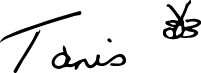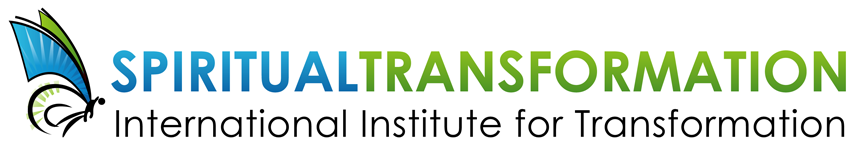# APRIL 2018

## Happy Easter, and a joyous welcome to spring!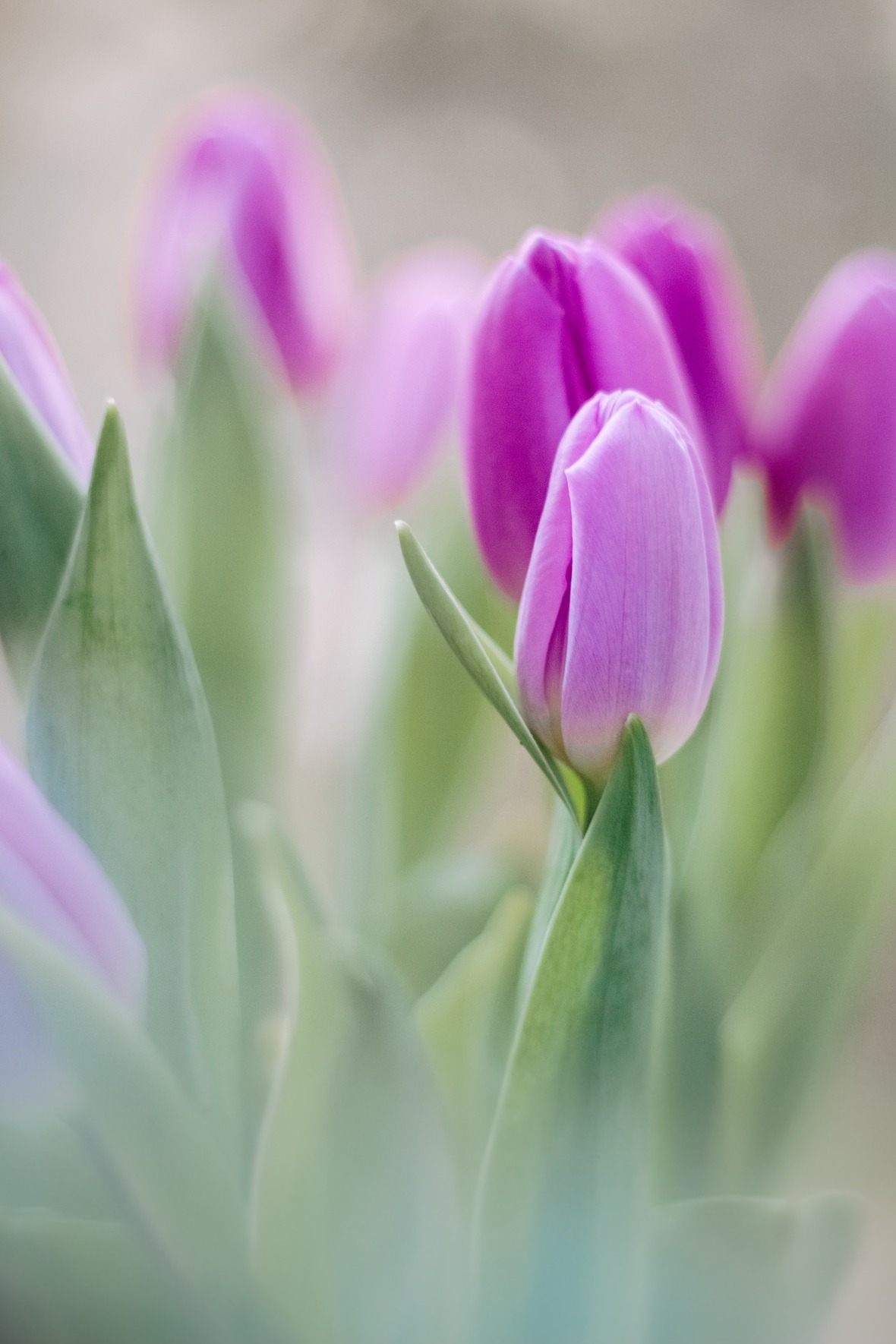Dear Friend,

New for April, a 20-minute Youtube video on "How to Become your Authentic Self", from a recent online interview that I did with Sigrid Van Heerwaarden.

I'm offering you a special discount on one of my books for April, Manifest Your Soul's Purpose. Enjoy!

I'm very excited about my annual July 15 to 21 meditation retreat at Diamond Heart. It is focused on a topic very dear to me, healing with the body elemental. Early bird pricing is on now until May 1st, and I do hope to see some of you here this summer.

The Tanis Helliwell website has had a facelift. Many thanks to Melany Hallam for her hard work on the redesign! I would love to hear what you think.

 table div table+table+table+table+table div table{width:100%;padding:0}table div table+table+table+table+table div table img{width:96.23%;padding:0;float:none}table div table+table+table+table+table div table td{width:100%;padding:0 1.88% 18px}/* styles *//* styles */ “Easter: The only time it's okay to put all of your eggs in one basket!" ― Unknown
 table div table+table+table+table+table+table+table div table{width:100%;padding:0}table div table+table+table+table+table+table+table div table img{width:96.23%;padding:0;float:none}table div table+table+table+table+table+table+table div table td{width:100%;padding:0 1.88% 18px}/* styles */## Video: How to Become Your Authentic Self

 /* styles */ In this new video, I speak with Sigrid Van Heerwaarden on “How to Become your Authentic Self". Sigrid is based in Holland and has a wonderful website called Your Best Life Starts Now: https://www.yourbestlifestartsnowseries.com/ Such an excellent interview with Sigrid and very worthwhile watching for anyone struggling to be true to themselves. The direct link to the Youtube video can be found here: https://youtu.be/bBxK6RFTV6c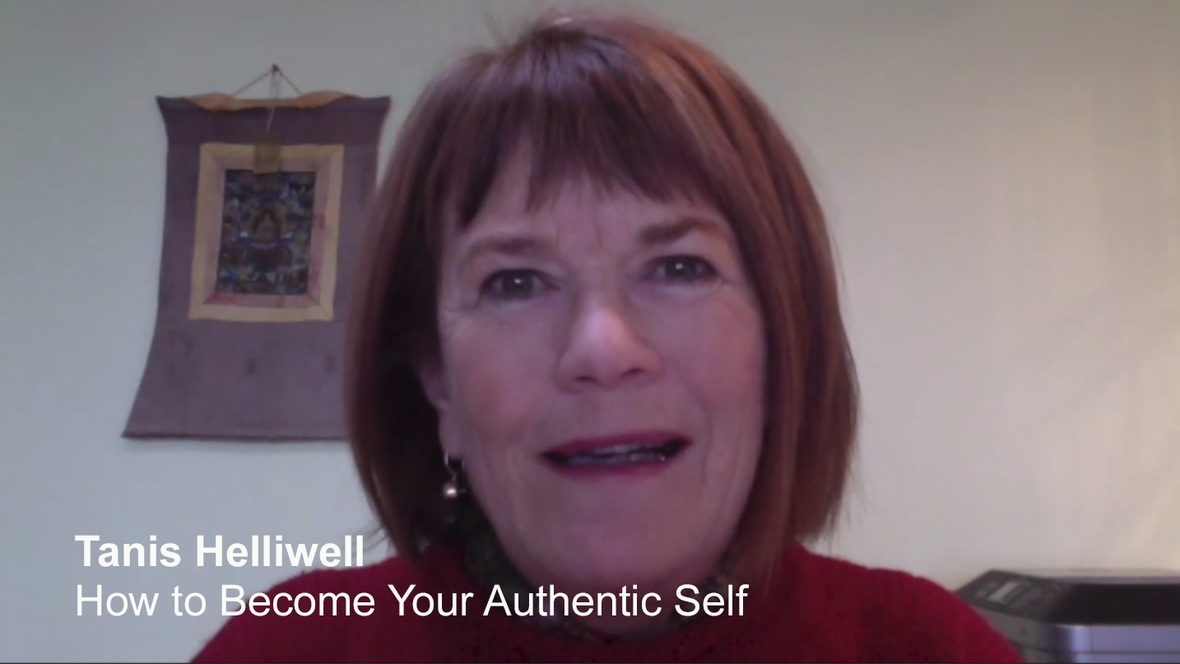Click on the image above to view the Youtube video, or copy and paste the direct link here: https://youtu.be/bBxK6RFTV6c
 table div table+table+table+table+table+table+table+table+table+table+table div table{width:100%;padding:0}table div table+table+table+table+table+table+table+table+table+table+table div table img{width:96.23%;padding:0;float:none}table div table+table+table+table+table+table+table+table+table+table+table div table td{width:100%;padding:0 1.88% 18px}/* styles */# 10% off for the Month of April

## The Essential Guide for Life and Work

Make a difference in your life, work and world! Manifest Your Soul’s Purpose assists you to live your highest potential. Tanis Helliwell guides you in seeing how to apply spiritual truths in your everyday life. This book helps to:

▪ Reprogram Yourself for Success
▪ Master the Material World
▪ Cultivate People who Feed Your Soul
▪ Live in Harmony with Natural Cycles
▪ Walk the Path of Heart
 ▪ Reprogram Yourself for Success
 ▪ Master the Material World
 ▪ Cultivate People who Feed Your Soul
 ▪ Live in Harmony with Natural Cycles
 ▪ Walk the Path of Heart

Manifest Your Soul’s Purpose: The essential guide for life and work will assist you to balance your life by discovering and acting upon your soul’s needs and by finding more meaning in your work.

## Read an excerpt from the book by choosing "Look Inside" on Amazon.com HERE.

 table div table+table+table+table+table+table+table+table+table+table+table+table+table+table+table+table div table{width:100%;padding:0}table div table+table+table+table+table+table+table+table+table+table+table+table+table+table+table+table div table img{width:96.23%;padding:0;float:none}table div table+table+table+table+table+table+table+table+table+table+table+table+table+table+table+table div table td{width:100%;padding:0 1.88% 18px}/* styles */table div table+table+table+table+table+table+table+table+table+table+table+table+table+table+table+table+table+table div table{width:100%;padding:0}table div table+table+table+table+table+table+table+table+table+table+table+table+table+table+table+table+table+table div table img{width:96.23%;padding:0;float:none}table div table+table+table+table+table+table+table+table+table+table+table+table+table+table+table+table+table+table div table td{width:100%;padding:0 1.88% 18px}/* styles */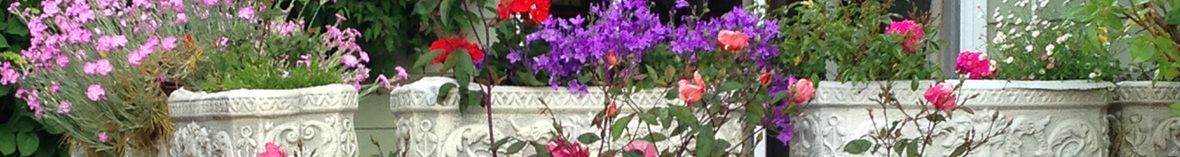# Early Bird registration now open!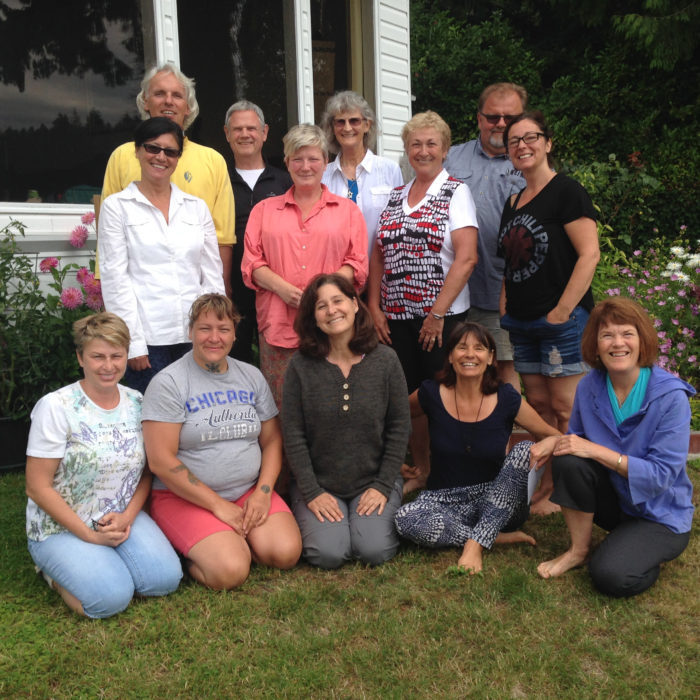Happy memories from the 2017 retreat.

## with Tanis Helliwell

Come to this meditation retreat to release, relax, and rejuvenate your heart and soul. Come bathe in the beauty of nature. Your heart will be grateful and your soul satisfied.

Enjoy delicious local and organic food during your stay. You will also have time to enjoy beach walks, guided hikes, swimming and kayaking, and an opportunity to see resident eagles, seals and sea lions.

Our Body Elemental has been with us since the beginning of time. It is time to work together to bring our body to optimum health. Develop a deeper relationship with your body elemental—your body consciousness—to diagnose causes of physical, emotional, mental weaknesses and co-create with it for optimal health.

▪ Learn about the mental and emotional causes of illness
▪ Heal fears that create energy blocks in your cellular-etheric body to increase your frequency
▪ Discover genetic and ancestral patterns to heal your family lineage
▪ Exercises to dissolve negative thoughtforms that cause illness and pain
▪ Find out how the mind and heart program the body
▪ Learn heart meditations to create harmonious resonance and entrainment for all systems.
▪ Practice techniques with others to remove energy blocks
▪ How self-healing leads to healing of others and the Earth
 ▪ Learn about the mental and emotional causes of illness
 ▪ Heal fears that create energy blocks in your cellular-etheric body to increase your frequency
 ▪ Discover genetic and ancestral patterns to heal your family lineage
 ▪ Exercises to dissolve negative thoughtforms that cause illness and pain
 ▪ Find out how the mind and heart program the body
 ▪ Learn heart meditations to create harmonious resonance and entrainment for all systems.
 ▪ Practice techniques with others to remove energy blocks
 ▪ How self-healing leads to healing of others and the Earth

Daily silent meditation, complimentary 1-hour private healing session, spiritual energization exercises, labyrinth, etheric clearing techniques.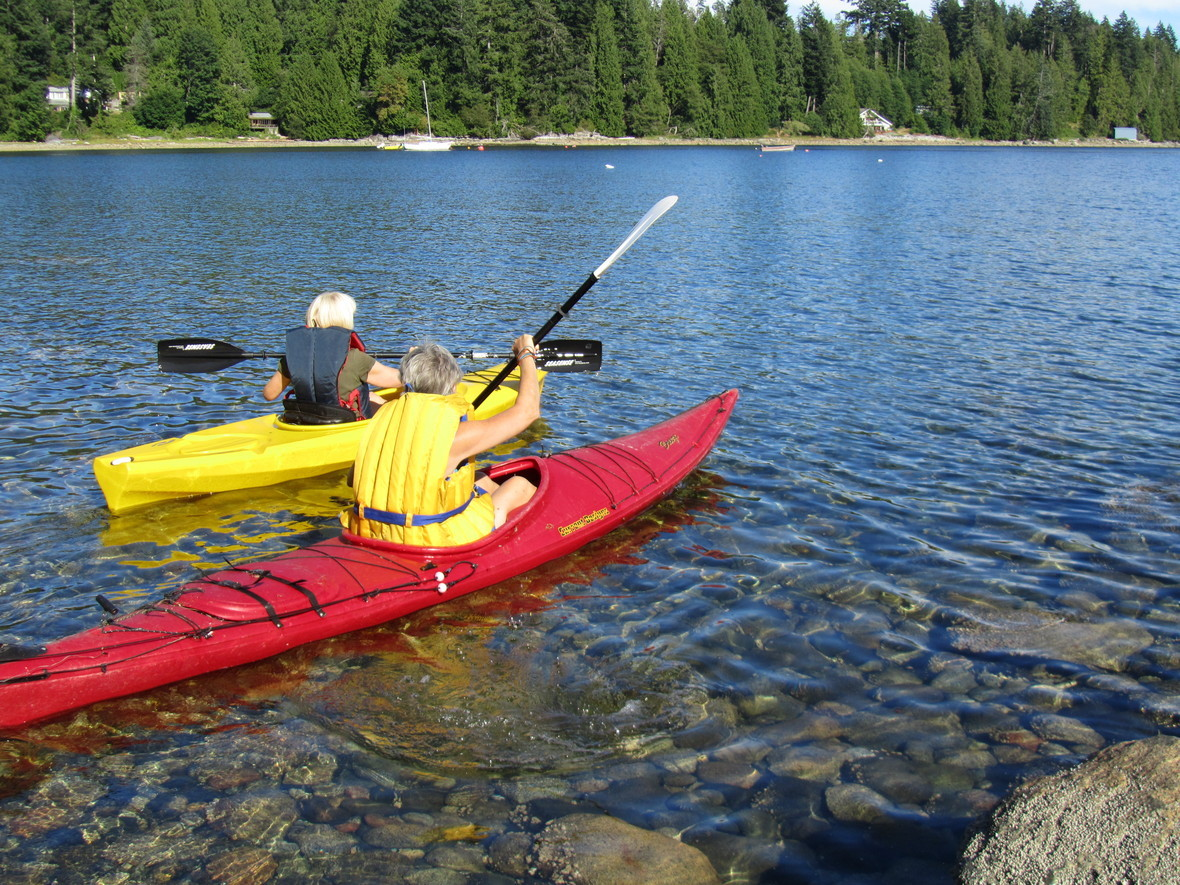Phone: (011)778-808-6101

Cost: \$695+ GST early bird until May 1st, \$795+ GST afterward May 1st. Accommodation and meals extra.

Location: Diamond Heart Retreat Center is beside the ocean offering swimming, kayaking, beach roaming, and forest walks and gardens.

Register early as space limited.

 table div table+table+table+table+table+table+table+table+table+table+table+table+table+table+table+table+table+table+table+table+table+table+table+table+table+table div table{width:100%;padding:0}table div table+table+table+table+table+table+table+table+table+table+table+table+table+table+table+table+table+table+table+table+table+table+table+table+table+table div table img{width:96.23%;padding:0;float:none}table div table+table+table+table+table+table+table+table+table+table+table+table+table+table+table+table+table+table+table+table+table+table+table+table+table+table div table td{width:100%;padding:0 1.88% 18px}/* styles */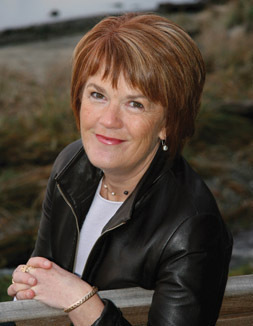Tanis Helliwell, M.Ed.,is a leading-edge psychotherapist, well-known for working with the body elemental to heal physical, emotional and mental traumas and patterns. Tanis teaches her techniques internationally to groups of psychiatrists, physicians, psychotherapists and other healing practitioners.

 table div table+table+table+table+table+table+table+table+table+table+table+table+table+table+table+table+table+table+table+table+table+table+table+table+table+table+table+table div table{width:100%;padding:0}table div table+table+table+table+table+table+table+table+table+table+table+table+table+table+table+table+table+table+table+table+table+table+table+table+table+table+table+table div table img{width:96.23%;padding:0;float:none}table div table+table+table+table+table+table+table+table+table+table+table+table+table+table+table+table+table+table+table+table+table+table+table+table+table+table+table+table div table td{width:100%;padding:0 1.88% 18px}/* styles */## 2018 European Workshops with Tanis

 table div table+table+table+table+table+table+table+table+table+table+table+table+table+table+table+table+table+table+table+table+table+table+table+table+table+table+table+table+table+table div table td,table.module-29{width:100%;padding:0}table div table+table+table+table+table+table+table+table+table+table+table+table+table+table+table+table+table+table+table+table+table+table+table+table+table+table+table+table+table+table div table{width:100%;float:none;margin-left:auto;margin-right:auto;padding:0}table div table+table+table+table+table+table+table+table+table+table+table+table+table+table+table+table+table+table+table+table+table+table+table+table+table+table+table+table+table+table div table a{border:0 none;text-decoration:none}table div table+table+table+table+table+table+table+table+table+table+table+table+table+table+table+table+table+table+table+table+table+table+table+table+table+table+table+table+table+table div table img{width:100%!important;border:0 none;text-decoration:none}/* styles */
 /* styles */ Join me this spring and summer at one (or several!) of my workshops offered across Europe. A strong focus this year is on Healing with the Body Elemental, and I am leading a 7-day Healing with the Body Elemental meditation retreat at Diamond Heart in BC, Canada from July 15-21, 2018. It would be lovely to see you!
 table div table+table+table+table+table+table+table+table+table+table+table+table+table+table+table+table+table+table+table+table+table+table+table+table+table+table+table+table+table+table+table+table+table div table{width:100%;padding:0}table div table+table+table+table+table+table+table+table+table+table+table+table+table+table+table+table+table+table+table+table+table+table+table+table+table+table+table+table+table+table+table+table+table div table img{width:96.23%;padding:0;float:none}table div table+table+table+table+table+table+table+table+table+table+table+table+table+table+table+table+table+table+table+table+table+table+table+table+table+table+table+table+table+table+table+table+table div table td{width:100%;padding:0 1.88% 18px}/* styles */## New look for Tanis Helliwell website!

 /* styles */ My Tanis Helliwell website has been redesigned and now has a gorgeous new look! I do hope that you enjoy the changes, and find them useful. Many thanks to Melany Hallam for her hard work and creative talent!
 table div table+table+table+table+table+table+table+table+table+table+table+table+table+table+table+table+table+table+table+table+table+table+table+table+table+table+table+table+table+table+table+table+table+table+table+table div table td,table.module-35{width:100%;padding:0}table div table+table+table+table+table+table+table+table+table+table+table+table+table+table+table+table+table+table+table+table+table+table+table+table+table+table+table+table+table+table+table+table+table+table+table+table div table{width:100%;float:none;margin-left:auto;margin-right:auto;padding:0}table div table+table+table+table+table+table+table+table+table+table+table+table+table+table+table+table+table+table+table+table+table+table+table+table+table+table+table+table+table+table+table+table+table+table+table+table div table a{border:0 none;text-decoration:none}table div table+table+table+table+table+table+table+table+table+table+table+table+table+table+table+table+table+table+table+table+table+table+table+table+table+table+table+table+table+table+table+table+table+table+table+table div table img{width:100%!important;border:0 none;text-decoration:none}/* styles */
 table div table+table+table+table+table+table+table+table+table+table+table+table+table+table+table+table+table+table+table+table+table+table+table+table+table+table+table+table+table+table+table+table+table+table+table+table+table div table{width:100%;padding:0}table div table+table+table+table+table+table+table+table+table+table+table+table+table+table+table+table+table+table+table+table+table+table+table+table+table+table+table+table+table+table+table+table+table+table+table+table+table div table img{width:96.23%;padding:0;float:none}table div table+table+table+table+table+table+table+table+table+table+table+table+table+table+table+table+table+table+table+table+table+table+table+table+table+table+table+table+table+table+table+table+table+table+table+table+table div table td{width:100%;padding:0 1.88% 18px}/* styles */table div table+table+table+table+table+table+table+table+table+table+table+table+table+table+table+table+table+table+table+table+table+table+table+table+table+table+table+table+table+table+table+table+table+table+table+table+table+table+table+table+table div table{width:100%;padding:0}table div table+table+table+table+table+table+table+table+table+table+table+table+table+table+table+table+table+table+table+table+table+table+table+table+table+table+table+table+table+table+table+table+table+table+table+table+table+table+table+table+table div table img{width:96.23%;padding:0;float:none}table div table+table+table+table+table+table+table+table+table+table+table+table+table+table+table+table+table+table+table+table+table+table+table+table+table+table+table+table+table+table+table+table+table+table+table+table+table+table+table+table+table div table td{width:100%;padding:0 1.88% 18px}/* styles */